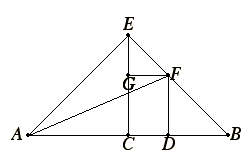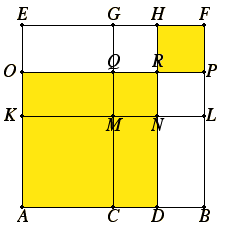Proposition 9

If a straight line is cut into equal and unequal segments, then the sum of the squares on the unequal segments of the whole is double the sum of the square on the half and the square on the straight line between the points of section.

Let a straight line AB be cut into equal segments at C, and into unequal segments at D.

I say that the sum of the squares on AD and DB is double the sum of the squares on AC and CD.Draw CE from C at right angles to AB, and make it equal to either AC or CB. Join EA and EB. Draw DF through D parallel to EC and FG through F parallel to AB. Join AF.

I.5

Then, since AC equals CE, the angle EAC also equals the angle AEC.

I.32

And, since the angle at C is right, the sum of the remaining angles EAC and AEC equals one right angle.

And they are equal, therefore each of the angles CEA and CAE is half of a right angle.

For the same reason each of the angles CEB and EBC is also half of a right angle, therefore the whole angle AEB is right.

And, since the angle GEF is half of a right angle, and the angle EGF is right, for it equals the interior and opposite angle ECB, the remaining angle EFG is half of a right angle. Therefore the angle GEF equals the angle EFG, so that the side EG also equals GF.

Again, since the angle at B is half of a right angle, and the angle FDB is right, for it is again equal to the interior and opposite angle ECB, the remaining angle BFD is half of a right angle. Therefore the angle at B equals the angle DFB, so that the side FD also equals the side DB.

Now, since AC equals CE, the square on AC also equals the square on CE, therefore the sum of the squares on AC and CE is double the square on AC.

I.47

But the square on EA equals the sum of the squares on AC and CE, for the angle ACE is right, therefore the square on EA is double the square on AC.

Again, since EG equals GF, the square on EG also equals the square on GF. Therefore the sum of the squares on EG and GF is double the square on GF.

I.47

But the square on EF equals the sum of the squares on EG and GF, therefore the square on EF is double the square on GF.

I.34

But GF equals CD, therefore the square on EF is double the square on CD.

But the square on EA is also double of the square on AC, therefore the sum of the squares on AE and EF is double the sum of the squares on AC and CD.

I.47

And the square on AF equals sum of the squares on AE and EF, for the angle AEF is right. Therefore the square on AF is double the sum of the squares on AC and CD.

I.47

But the sum of the squares on AD and DF equals the square on AF, for the angle at D is right, therefore the sum of the squares on AD and DF is double the sum the squares on AC and CD.

And DF equals DB.

Therefore the sum of the squares on AD and DB is double the sum of the squares on AC and CD.

Therefore if a straight line is cut into equal and unequal segments, then the sum of the squares on the unequal segments of the whole is double the sum of the square on the half and the square on the straight line between the points of section.

Q.E.D.

Guide

Outline of the proof

Start with the given line AB bisected at C and cut at another point D. The construction will result in a line AF whose square is simultaneously equal to both AD2 + DB2 and 2(AC2+CD2).

Draw CE at right angles to AB and equal to half of it. Finish the diagram by drawing parallel lines and connecting points. That results in four isosceles right triangles, ACE, ECB, EGF, and FDB, as well as two other right triangles, AEB and AEF. Then,

 AF2 = AE2 + EF2 = (AC2 + CE2) + (EG2 + GF2) = 2(AC2+CD2)
But also,

Thus, AD2 + DB2 = 2(AC2+CD2), as desired.

An alternate cut-and-paste proof

 Up until this proposition, Euclid has only used cut-and-paste proofs, and such a proof can be made for this proposition as well. It would start with the same line AB bisected at C and also cut at D. Then lines at right angles and parallel to line AB would be constructed to make squares and rectangles of various sizes. The goal is to show that AD2 + DB2 = 2(AC2 + CD2). The left hand side of the equation is displayed to the right as the two squares AR plus RF. Cut out the rectangle KQ and move it to the right to cover the rectangle MP. Also move the rectangle CN up to the rectangle MH. Now the upper right square MF is completely covered once, with two extra coverings of the small square MR. That is, two squares of size AC2 are covered as well as two squares of size CD2. The two sides of the equation are, therefore, equal.Interpretations of the proposition

The equation, AD2 + DB2 = 2(AC2+CD2), can be interpreted in various ways depending on which information are taken as given. These interpretations are more of an aid to the modern reader than as intrinsic aspects of the proposition, since they are interpretations in modern symbolic algebra.

For instance, when AC and CB are set to y while CD is set to z, then the algebraic identity

(y + z)2 + (y – z)2 = 2 (y2 + z2)

results.

Alternatively, when AC and CB are set to y while BC is set to x,, then we get the identity

(2yx)2 + x2 = 2 (y2 + (yx)2)

There are yet other interpretations. This proposition is used in Book X to prove a lemma for X.60, and in that lemma, w = AD and x = DB are given first, so that

w2 + x2 = 2 (((w + x)/2)2 + ((wx)/2)2)